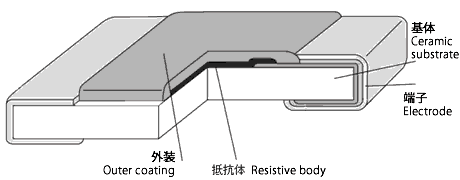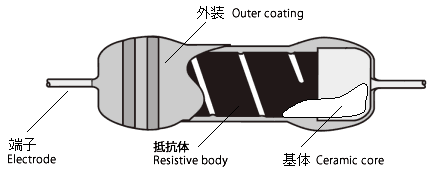# Products# Basic of Resistors

## What is Resistor?

To understand a resistor, the important thing is to understand Ohm's Law, first of all.
This is the basic knowledge.
Ohm's Law means [the current what flows in to an electric conduction object is proportional to the voltage of both end of it] and defines the relationships between (E) voltage, (I) current, and (R) resistance as below.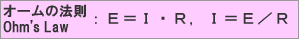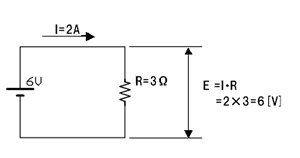R is proportionality coefficient and called resistance(electric resistance). Resistance volume code is R, unit code is Ω(ohm), and electronic symbol is expressed as below.

[Electronic symbols of resistor］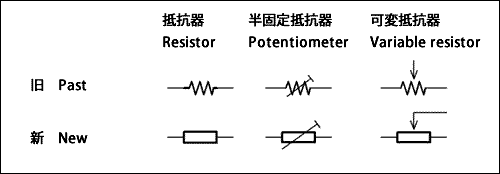If the resistance is higher, the current will be difficult to flow, but easy to flow if the resistance is lower. In other words, resistor works to keep the current flowed to a circuit stable and to change it if necessary. Resistor also works to decrease the voltage or divide the voltage.
Resistor is thus indispensable product for one of the fundemental components of electric circuit, as capacitors and inductors.

## Material construction and role

Resistor is basically composed of following four elements.

### Base substrate: holds resistive body and terminals

Alumina type insulators are most used for base substrate of resistors.
We have to select suitable material(thermal conductivity, thermal expansion of coefficient, mechanical strength) according to the purpose of resistor.

### Resistive body: part that determines basic features and performances of resistor

Electric resistance
Here is the substance as shown in right
When
Section area[cm2]：S
Length[cm]：L
Specific resistance of the substance[Ω・cm]：ρ
Electric resistance R of this substance would be R＝ρ・L ／ S [Ω]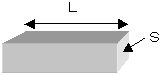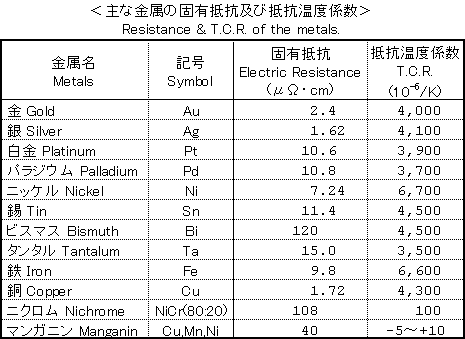### Terminal:Electrically and mechanically connects the resistor and the board (circuit pattern).

The electrical connection point of the resistor, where voltage and current are applied. The structure and material are configured so that it does not affect the characteristics of the resistor.

Select a resistor with a terminal structure and shape suitable for the mounting method. Surface mount components (SMD) are now the mainstream in order to improve the mounting density and reduce the mounting cost..

### Coating: Protects the resistive element and base substrate from the ambient air and mechanical stress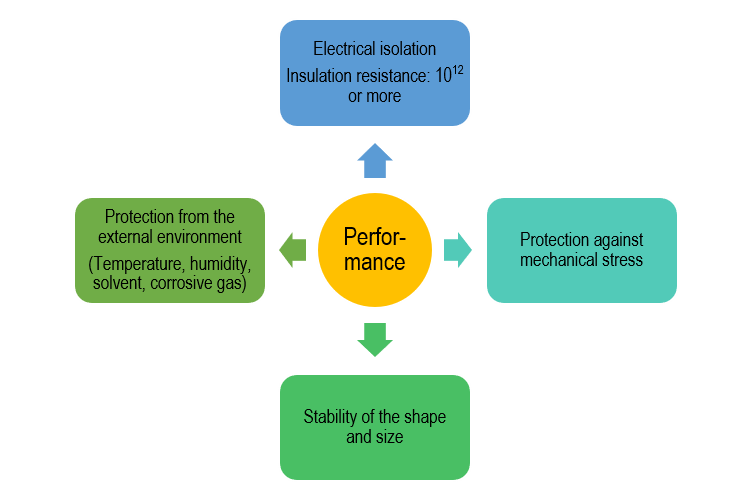### Construction example of resistor

• Flat Chip Resistor